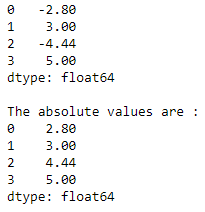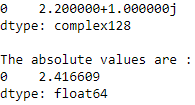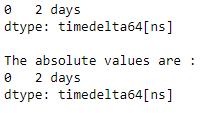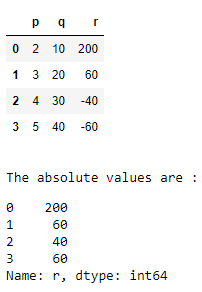Related Articles

# Get the absolute values in Pandas

• Difficulty Level : Medium
• Last Updated : 18 Aug, 2020

Let us see how to get the absolute value of an element in Python Pandas. We can perform this task by using the `abs()` function. The abs() function is used to get a Series/DataFrame with absolute numeric value of each element.

Syntax : Series.abs() or DataFrame.abs()
Parameters : None
Returns : Series/DataFrame containing the absolute value of each element.

Example 1 : Absolute numeric values in a Series.

 `# import the library``import` `pandas as pd`` ` `# create the Series``s ``=` `pd.Series([``-``2.8``, ``3``, ``-``4.44``, ``5``])``print``(s)`` ` `# fetching the absolute values``print``(``"\nThe absolute values are :"``)``print``(s.``abs``())`

Output :Example 2 : Absolute numeric values in a Series with complex numbers.

 `# import the library``import` `pandas as pd`` ` `# create the Series``s ``=` `pd.Series([``2.2` `+` `1j``])``print``(s)`` ` `# fetching the absolute values``print``(``"\nThe absolute values are :"``)``print``(s.``abs``())`

Output :Example 3 : Absolute numeric values in a Series with a Timedelta element.

 `# import the library``import` `pandas as pd`` ` `# create the Series``s ``=` `pd.Series([pd.Timedelta(``'2 days'``)])``print``(s)`` ` `# fetching the absolute values``print``(``"\nThe absolute values are :"``)``print``(s.``abs``())`

Output :Example 4 : Fetching the absolute values from a DataFrame column.

 `# import the library``import` `pandas as pd`` ` `# create the DataFrame``df ``=` `pd.DataFrame({``'p'` `: [``2``, ``3``, ``4``, ``5``],``                   ``'q'` `: [``10``, ``20``, ``30``, ``40``],``                   ``'r'` `: [``200``, ``60``, ``-``40``, ``-``60``]})``display(df)`` ` `# fetching the absolute values``print``(``"\nThe absolute values are :"``)``display(df.r.``abs``())`

Output :Attention geek! Strengthen your foundations with the Python Programming Foundation Course and learn the basics.

To begin with, your interview preparations Enhance your Data Structures concepts with the Python DS Course. And to begin with your Machine Learning Journey, join the Machine Learning – Basic Level Course

My Personal Notes arrow_drop_up